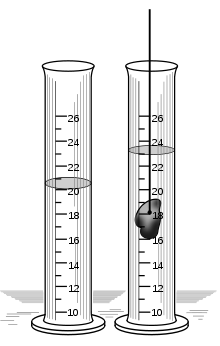# Displacement (fluid)

Displacement (fluid)Two graduated cylinders containing water, one with a rock submerged in it, showing the increased water level due to displacement

In fluid mechanics, displacement occurs when an object is immersed in a fluid, pushing it out of the way and taking its place. The volume of the fluid displaced can then be measured, as in the illustration, and from this the volume of the immersed object can be deduced (the volume of the immersed object will be exactly equal to the volume of the displaced fluid).

An object that sinks displaces an amount of fluid equal to the object's volume. Thus buoyancy is expressed through Archimedes' principle, which states that the weight of the object is reduced by its volume multiplied by the density of the fluid. If the weight of the object is less than this displaced quantity, the object floats; if more, it sinks. The amount of fluid displaced is directly related (via Archimedes' Principle) to its weight.

In the case of an object that sinks (is totally submerged), the volume of the object is displaced. In the case of an object that floats, the amount of fluid displaced will be equal in weight to the displacing object.

## Applications of displacement

Commonly called water displacement, this method can be used to measure the volume of a solid object, even if its form is not regular. Several methods of such measuring exist. In one case the increase of water level is registered as the object is immersed into the water. In the second case the object is immersed into a vessel full of water, causing it to overflow. Then the overflown water is collected and its volume measured. In the third case the object is suspended under the surface of the water and the increase of weight of the vessel is measured. It is equivalent to the weight of the amount of water that has the volume equal to the one of the suspended object.

The weight of an object or substance can be measured by floating a sufficiently buoyant receptacle in the cylinder and noting the water level. After placing the object or substance in the receptacle, the difference in weight of the water level volumes will equal the weight of the object.It is a method

Wikimedia Foundation. 2010.

### См. также в других словарях:

• Displacement — may refer to: Contents 1 Physical sciences 1.1 Physics 1.2 Engineering …   Wikipedia

• Fluid statics — (also called hydrostatics) is the science of fluids at rest, and is a sub field within fluid mechanics. The term usually refers to the mathematical treatment of the subject. It embraces the study of the conditions under which fluids are at rest… …   Wikipedia

• Displacement thickness — is the distance by which a surface would have to be moved parallel to itself towards the reference plane in an ideal fluid stream of velocity u 0 to give the same mass flow as occurs between the surface and the reference plane in a real fluid.In… …   Wikipedia

• displacement — [dis plās′mənt, dis′plās′mənt] n. 1. a displacing or being displaced 2. a) the weight or volume of a fluid displaced by a floating object; specif., the weight of water, in long tons, displaced by a ship b) the volume displaced by a stroke of a… …   English World dictionary

• fluid mechanics — an applied science dealing with the basic principles of gaseous and liquid matter. Cf. fluid dynamics. [1940 45] * * * Study of the effects of forces and energy on liquids and gases. One branch of the field, hydrostatics, deals with fluids at… …   Universalium

• displacement — /dis plays meuhnt/, n. 1. the act of displacing. 2. the state of being displaced or the amount or degree to which something is displaced. 3. Physics. a. the displacing in space of one mass by another. b. the weight or the volume of fluid… …   Universalium

• displacement — 1. Removal from the normal location or position. 2. The adding to a fluid (particularly a gas) in an open vessel one of greater density whereby the first is expelled. 3. In chemistry, a change in which one element, radical, or mole …   Medical dictionary

• displacement pump — noun : a pump (as an air lift or pulsometer that raises or transfers a fluid by direct displacement with no transformation of the kinetic energy due to the fluid s motion into potential energy due to pressure …   Useful english dictionary

• displacement — noun Date: 1611 1. the act or process of displacing ; the state of being displaced 2. a. the volume or weight of a fluid (as water) displaced by a floating body (as a ship) of equal weight b. the difference between the initial position of… …   New Collegiate Dictionary

• Displacement Volumetric Meter — A Displacement Volumetric Meter is a device which measures total volume. It can operate on the principle of the fluid sweeping out a fixed calibrated volume, which produces a rotation of a calibrated gauge. By maintaining constant conditions and… …   Wikipedia

### Поделиться ссылкой на выделенное

##### Прямая ссылка:
Нажмите правой клавишей мыши и выберите «Копировать ссылку»